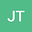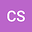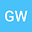Approximation factor of the piecewise linear functions in Mamdani fuzzy systems and its realization process
•••chunfeng suo
Tonghua Normal University
Author ProfileGuijun Wang
Tianjin Normal University
Author Profile## Abstract

A piecewise linear function is not only an extension of a segmented linear function of one variable in the case of multivariate variables, but also an important bridge to study the approximation of a continuous function by Mamdani and Takagi-Sugeno fuzzy systems. In this paper, the concepts of a piecewise linear function and subdivision number are introduced in hyperplane, the analytic expression of the piecewise linear function is given by matrix determinant, and a new definition of the approximation factor is first proposed by m-mesh subdivision. Secondly, by the method of generating small polyhedron from three-dimensional cube, the change rule of vertex coordinates of n-dimensional subdivision polyhedron is studied, the vertex coordinates of small polyhedron are obtained by rotating component coordinates of their respective coordinate axes. Furthermore, the calculation methods of algebraic cofactor and matrix norm for the corresponding determinant are given. Finally, according to the method of solving algebraic cofactors and matrix norms, it is proved that the approximation factor has nothing to do with the subdivision number, but the approximation precision has something to do with the subdivision number. In addition, the realization process of a specific binary piecewise linear function approaching a continuous function according to infinite norm in two dimensions space is given by an example.